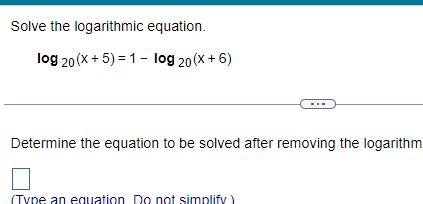Question:

# Solve the logarithmic equation log 20 x 5 1 log 20 X 6

Last updated: 11/21/2023Solve the logarithmic equation log 20 x 5 1 log 20 X 6 Determine the equation to be solved after removing the logarithm Type an equation Do not simplify